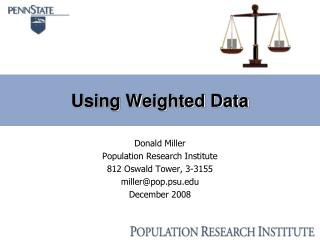DownloadDownload PresentationUsing Weighted Data

# Using Weighted Data

Download Presentation## Using Weighted Data

- - - - - - - - - - - - - - - - - - - - - - - - - - - E N D - - - - - - - - - - - - - - - - - - - - - - - - - - -
##### Presentation Transcript

1. Using Weighted Data Donald Miller Population Research Institute 812 Oswald Tower, 3-3155 miller@pop.psu.edu December 2008

2. Review of David Johnson’s Presentation • A population weight (pweight) is a variable which indicates how many people (in the population of interest) an observation will count in a statistical procedure. This is different from a frequency weight (fweight), which indicates a row of a dataset actually represents more than one observation. • Weights can be used to correct for design (over- and under- sampling), and for non-response bias. • Most software packages treat pweights properly (a notable exception is SPSS outside of complex survey package). • To create a pweight, use either a “raking”-type algorithm, or a logistic regression.

3. How to use Population Weights • SAS: Use the “weight” statement in procedures; this is a population weight: proc logistic data=mydata descending; model finished=age cs_educ sex race_white a1b a2b a3b a4b a5b a6b; weight pwgt_variable; run; • Stata: Use the “pweight” option (you can use “pw”): regress y x1 x2 x3 [pweight=pwgt_variable]

4. Raking 1: Select Census Data • Choose a census dataset (CPS, ACS, etc.), and which variables you will use in your “raking model”. These are usually demographics variables (age, race, education, gender). • You will need to recode your survey variables and/or the census variables so the response categories match. This might require grouping some values together. • Match the year of the survey with the census data. If you have 2006 survey data, use the 2006 census data. • Match the physical area as closely as possible. For example, the ACS uses PUMA codes (basically county-level data). Select only the PUMA codes of the area of interest. • You should probably do some simple descriptives / frequencies to compare survey to census. Remember the ACS already has a weight (PWGTP).

5. Raking 2: Frequencies (Census data) • Construct 1-way frequency counts for every variable in the raking model. You need a dataset for each variable, with “mrgtotal” being the counts. SAS code example (do this for gender, race, etc.): proc freq data=acs.acs_myarea_recoded; table cs_educ /list missing out=cs_educ; weight PWGTP; run; data cs_educ; set cs_educ; rename COUNT=mrgtotal; run;

6. Raking 3: Raking Macro (SAS) Izrael, etc. has provided a SAS Macro (RAKINGE) to do the main raking procudure. This is introduced in Paper 258-25, from SAS SUGI 25. This is available online from SAS at: http://www2.sas.com/proceedings/sugi25/25/st/25p258.pdf Various improvements were made to macro and introduced in Paper 207-29, from SAS SUGI 29. This is available online from SAS at: http://www2.sas.com/proceedings/sugi29/207-29.pdf I uploaded the (corrected version of the) RAKINGE macro here: http://help.pop.psu.edu/help-by-statistical-method/weighting

7. Raking 4: Raking Macro (SAS) You will need to save this macro, edit it slightly, and run it. The vast majority of the code you will never touch. Towards the top of the program you will need to change these lines: %macro rakinge (inds=INPUTDATASETNAME, outds=OUTPUTDATASETNAME, ... outwt=NEW_PWEIGHT_VARIABLE_NAME, ... varlist=LIST OF VARIABLES IN RAKING MODEL, numvar=4,

8. Normalized Weight If the raking macro does not converge, look at the frequencies (for census and survey) again. You may need to collapse some categories, or change the convergence criterion in the raking macro (you can control this with the TRMPCT= and NUMITER= options in the macro). You may wish to “normalize” the weight, so the sum of the weights for the dataset equal to a predetermined number N (either sample size or the area’s total population). To do this, calculate SW = the sum of the weights then multiply each weight value by N/SW.

9. Non-Response Bias 1 The probability to survey completion may differ with people of different characteristics (demographics, chronic conditions, etc.). To address this non-response bias, estimate a logistic regression model such as the following: FINISH = β0 + β1AGE + β2EDUCATION + β3FEMALE + β4WHITE + β5OTHER + ε Where FINISH is 1 if they finished the survey (0 otherwise). The next four values are from the raking model. The next value (OTHER, there can be more than one of these) are other variables which might explain non-response bias.

10. Non-Response Bias 2 For each respondent, the non-response bias weight is the reciprocal of the predicted probability of survey completion. It is treated as a weight, and should be multiplied with the raking weight to create a total weight. Sample SAS code (continued next slide): proc logistic data=area_weighted descending; class finish; model finish=age cs_educ sex race_white a1b; output out=logitresults p=p; weight pwgt; run; /* check output to see if significant */

11. Non-Response Bias 3 (SAS code continued): proc sort data=logitresults; by ID; run; data area_weighted2; /* merge in pred. prob. */ merge area_weighted logitresults; by ID; run; data area_weighted2; /* calculate non-resp wgt */ set area_weighted2; nonresp_wgt=1/p; total_wgt=pwgt*nonresp_wgt; run;

12. Stata / R I personally haven’t tried either of these yet, but raking packages exist for Stata and R: Stata: survwgt - you can get and install this using findit survwgt rake [pw] , by(varlist_raking_model) totvars(varlist_totals) { generate(pwgt) | replace } R: Rake package sraked_data <- simpleRake(unraked_data, pop_totals, “rake_var1”, “rakevar2”, ..., TRUE)